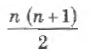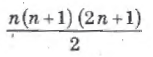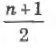# VAO 2014 June GE TNPSC Question Paper

## VAO 2014 June GE TNPSC Questions

1.
The average age of 50 students in 10th std class is 15 years. 10 more students are admitted a fresh in the class and the average age is increased by 0.5 years. The average age of the newly joined students is
A.
15
B.
16
C.
17
D.
18
D. 18
2.

What percentage of students does not like computer science?

 Subject No. of students Mathematics 6 Physics 12 Chemistry 15 Computer Science 9
A.

91%

B.

9%

C.

82%

D.

18%

C. 82%

3.
If the simple interest for an amount at 4% per annum for 3 years is Rs. 1,200. Find the compound interest at the same rate for the same amount for 2 years.
A.
Rs. 10,116
B.
Rs. 10,720
C.
Rs. 10,616
D.
Rs. 816
D. Rs. 816
4.

Which of the following is/are true?

(i) ax = bx => a = b

(ii) x/a =x/b => a = b

(iii) a = b => ax = bx

(iv) a + x = b + x => a = b

A.

(i), (ii), (iii) and (iv)

B.

(i), (iii) and (iv) only

C.

(i) and (iv) only

D.

(iii) and (iv) only

D. (iii) and (iv) only

5.
Two dice are thrown simultaneously. The proability of getting a doublet is
A.
1/36
B.
1/3
C.
1/6
D.
2/3
C. 1/6
6.

The mean of the first n natural numbers

A.B.C.D.

n2

C.7.
In a set of first 'x' natural numbers there 30 prime number. Find the number of composite numbers in the above set.
A.
x - 30
B.
30 - x
C.
x - 29
D.
x - 31
D. x - 31
8.
If '+' means 'x', '-' means '+', 'x' means '÷' and '÷' means '-' then the value of 84 x 28 + 8÷10 - 9
A.
23
B.
22
C.
30
D.
26
A. 23
9.

Consider the following statements.

I. The sun is a medium sized star

II. It is about two billion years old

III. It consist of almost entirely of hydrogen and helium

Which of the statements given above is/are correct?

A.

I only

B.

I and II

C.

I and III

D.

I, II and III

C. I and III

10.

Consider the following statements.

I. Forests grow lush in areas where there are conditions such as high temperature, low rainfall and fertile soils.

II. Forests grow lush in areas where there are conditions such as high temperature and heavy rainfall throughout the year and fertile soils.

Which of the statements given above is/are correct?

A.

I only

B.

II only

C.

I and II only

D.

I and II are wrong